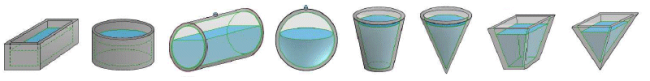# Tank Types and Different Shapes of TankThere are many different shapes and types of tank. Engineers often need to calculate the volume of material that is used to construct a tank, the weight of tank material, the capacity of the tank, the volume of fluid in a part filled tank of a particular shape, and the weight of fluid in a tank. Pipe Flow Advsior can calculate these properties for a range of common tanks types of different shapes and proportions:

• Rectangular Tanks
• Circular Tanks
• Cylindrical Tanks
• Cone Tanks or FrustrumTanks
• Hopper Tanks or Pyramid Tanks

## Tank Properties & Tank DimensionsPipe Flow Advisor has a separate calculation screen for each style of tank. It makes it easy to calculate tank properties, such as volume of material, tank capacity and tank weight. It can also calculate the volume and weight of fluid for part filled tanks, for a given height of fluid, which is not an easy calculation for tanks with sloping sides, horizontal cylinders and spherical tanks, where the relationship between fluid height and volume is not linear.

Another useful calculation that the software performs, is to estimate the tank emptying time, for flow under gravity. As the depth of fluid in the tank changes as flow is discharged, the head of fluid pressure changes and thus the emptying flow rate changes. By calculating the flow rate for a specific condition and iterating over time, the Pipe Flow Advisor software can calculate the overall tank emptying time and show the emptying flow rate at various time intervals, through to the time when the tank becomes empty.

Next: Tank Emptying Time Calculator

Secure Online PaymentsPipe Flow Software: Piping design, Pressure drop calculator, Flow rate calculator, Pump head calculations, Pump selection software.  Copyright © Pipe Flow Software 1997-2020